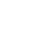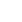# The Value of a Number

JRDAssist
Middle School and High School Teacher

The students will work in groups of 4-6 physically learning and reviewing place value.

##Length of Time: 1-2 Class Periods

### Common Core Alignment

CCSS.Math.Content.4.NBT.A.1 - Recognize that in a multi-digit whole number, a digit in one place represents ten times what it represents in the place to its right. For example, recognize that 700 ÷ 70 = 10 by applying concepts of place value and division.

## Objectives & Outcomes

The students will be able to identify the value of each digit in a given number from ones to millions.

## Materials Needed

Plain sheets of paper/cardboard, markers

Prepare ahead of time:  Example sheets of paper with numbers, some with commas; each place value written out on a sheet of the same size paper (ones, tens, hundreds, one-thousands, ten-thousands, etc.) to be used as “labels”; a 7 to 9-digit number with meaning.

## Procedure

### Opening to Lesson

• Display a 7 to 9-digit number for students to read aloud as a group or one student at a time
• Use a number with an interesting meaning (Distance from a planet to the Sun, etc.)
• Ask students if they can guess the significance of the number
• Allow students to identify the place value for each digit

### Body of Lesson

#### Modeling

• Hand out the example sheets of paper w/digits to several students
• Have those students create a number while standing in front of the room
• Call on students to read aloud the displayed number
• Give the labels to other students to display correctly
• Tel students they will be creating similar numbers in a group

#### Guided Practice

• Assign students to groups of 4-6
• Distribute blank sheets of paper/cardboard and markers
• Assign one student from each group to be the secretary to list at least 5 numbers the group will display to the class
• Instruct the students to create enough digits to display the numbers to the rest of the class
• Encourage students to work together and assure everyone in the group is able to read each number and identify the value of each digit (Some may not be able to do this, but the lesson will hopefully help those students understand place value better.)
• Encourage students to start out with smaller numbers and progress to larger numbers
• Once all groups are ready, create an order for each group to present the numbers in front of the classroom
• When a group first displays a number, the class will together read it aloud
• As each group presents, the teacher will then choose randomly seated students to label each digit’s place value by holding the label correctly under the number in the display (Do this one digit at a time.)
• Continue with the procedure until all groups have had a chance to display their created numbers

#### Independent Practice

• For homework, assign students a place-value worksheet, or have students find large numbers with significance to read aloud to the class the next day, sharing the information about the number

### Closing

Discuss the importance of understanding place value, why numbers have different values, etc.

## Assessment & Evaluation

Assess the in-class assignment or homework for understanding

## Modification & Differentiation

Students may work in larger groups.  Use the activity as a quiz/test for place value by eliminating the class discussion, each group having 2 or 3 turns to display a number to be identified and written out.  Extend the lesson to billions, limit to one millions, etc.  Do lesson outdoors or in the gym where there is more space.  Use different colored markers for places.

## Related Lesson Plans

##### Graph Smile

Pairs of students will survey other students in the school and then graph the results, as well as come to conclusions based on the information.

##### Comparing Decimals Game

Students will play a game in which they choose cards and choose the best place to put the number they have chosen in order to get the highest answer possible.  This should not be the first introduction to the topic.

##### Shape Up

This lesson will allow students to demonstrate knowledge of various grade appropriate shapes.

##### Scale It Up

This lesson will allow students to demonstrate knowledge use of scale.

##